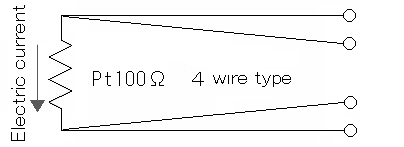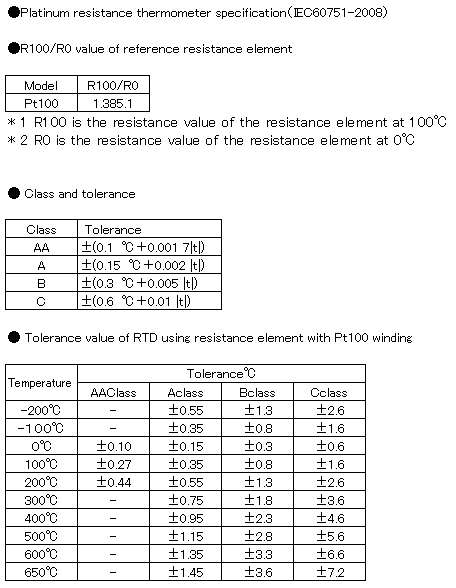Home / Air Blow Heater / Basic knowledge of Temperature controller / 2-2.Platinum resistance thermometer

# 2-2.Platinum resistance thermometer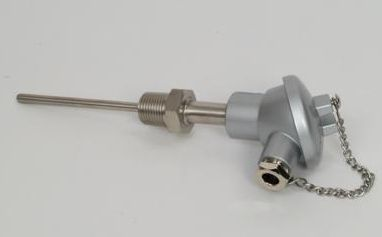A resistance temperature detector is a temperature sensor that utilizes the fact that the electrical resistivity of metal changes in proportion to temperature.
Metals increase resistance by about 0.3% when the temperature rises by 1K.
Although any metal can be used in principle, platinum (Pt) is generally used because the resistance change with temperature is constant and the rate of change is large.
The platinum resistor thermometer was invented in 1885 by Calende-Van Duesen.

The measurement method is to apply a constant current (1mA in general) to a metal resistor, measure the voltage with a measuring instrument, convert the resistance from Ohm’s law E = IR to the resistance value, and derive the temperature.

When writing this in the equation, use the temperature t (° C) for the resistivity ρ of the metal.

ρ＝ρ0（1＋αt）

ρ0 is the resistivity at 0 ° C, and α is the temperature coefficient of resistance. Since the extent to which the metal length l and cross-sectional area A change with temperature is extremely small, multiplying both sides of the above equation by l / A gives the following equation.

R＝R0（1＋αρ）

It is the platinum resistor that determines the temperature by measuring the resistance value of platinum (Pt) using this formula. Platinum resistor is a structure in which platinum with a diameter of several tens of μm is wound around glass or ceramics and put in a protective tube.

The temperature measurement range of the platinum resistance thermometer is about -200 to 500 ° C.

2-wire type
Although one conductor is connected to each end of the RTD element, it is not suitable for high-precision measurement because it is affected by the conductor resistance (Class A and sheathed resistor standard) (Not applicable to IEC60751-2008).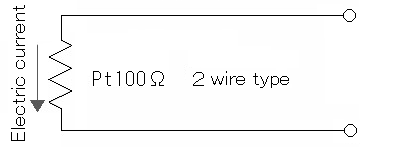3-wire type
Two conductors are connected to one end of the resistance thermometer element and one conductor is connected to the other end. This is the most widely used and highly reliable measurement method for industrial use, excluding the effect of conductor resistance.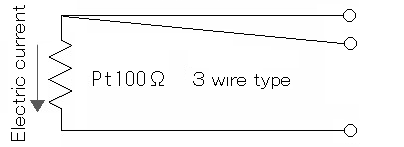4-wire type
Two conductors are connected to both ends of the resistance thermometer element, respectively, and it is suitable especially when high-precision measurement is required, excluding the effect of conductor resistance.